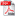# COMPARATIVE DISCUSSION ABOUT THE DETERMINING METHODS OF THE STRESSES IN PLANE SLABS

Autor/autori: Codrin PRECUPANU, Dan PRECUPANU, Ștefan OPREA

Abstract: In this paper it is presented the necessity of intense developing of the finite differences method, highlighted by the authors since the first Congress of Numerical Calculus in Bruxelles 1992, at the determining of the stress state in plane structural elements by comparison with finite elements method. We determine the normal stresses in a point of an octogonal plane slab subjected to a plane system of forces by finite differences method and finite elements method. It is easily established, that the calculus volume using the first method is much diminished comparative with the one that is necessary for using the second method. The difference between values obtained by the two methods are under 10%. Furthermore, in finite differences method the accuracy of the results can be easily increased if are used relations for expressing the derivatives with improved finite differences without reducing the spacing of the discretization network, which is another important benefit of this method. The applying of the finite differences method in problems defined on double conex domains offers a wide field of using this method, being distinguished as an engineering method with advantages of rapidity in determining the stresses in plane structural elements

Keywords: numerical methods, finite differences, finite elements, stresses, plane slabDOWNLOAD PDF